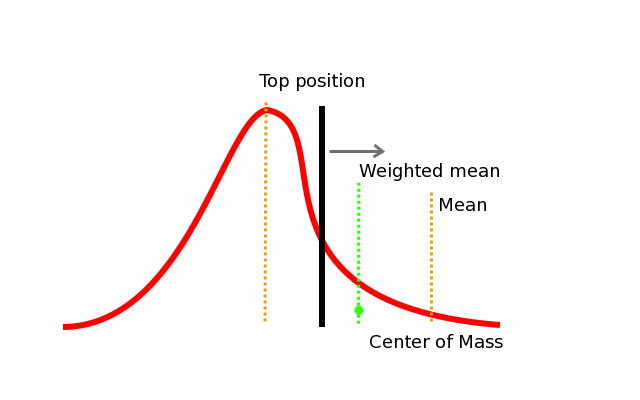# Emission wavelength

The emission wavelength is the WaveLength of the radiation emitted by the object being measured, coming from the fluorescent dyes. The fluorescent emission is caused by a radiation source with a given Excitation Wavelength.

What to set as an emission wavelength when a certain range of wavelength the socalled bandwidth is used or when long pass emission filters are applied during acquisition?

For the bandwidth situation the best setting would then be the weighted mean of the wavelengths, or the center of mass. This would set the emission wavelength between the mean and the top of the wavelength distribution. However, the weighted mean does not have much influence so setting the mean instead of the weighted mean is really good enough and very easy to calculate when using a (limited) bandwidth of fluophores.Visualization of the difference between wavelength positions for bandlimited emission filter.

For the long pass emission filters the mean is difficult to calculate, because the max is not known (to infinity), so here the weighted mean should be taken. Because the weight of the high wavelengths is very low, the setting should stay quite close to the minimum wavelength. For Huygens it is enough to estimate this value instead of really calculate it.Visualization of the difference between wavelength positions for long pass emission filter.